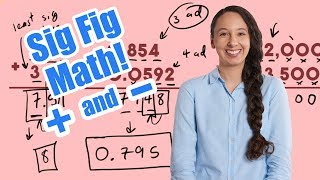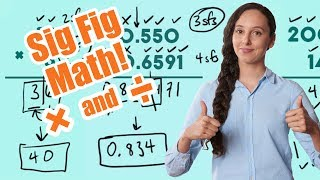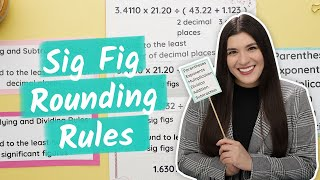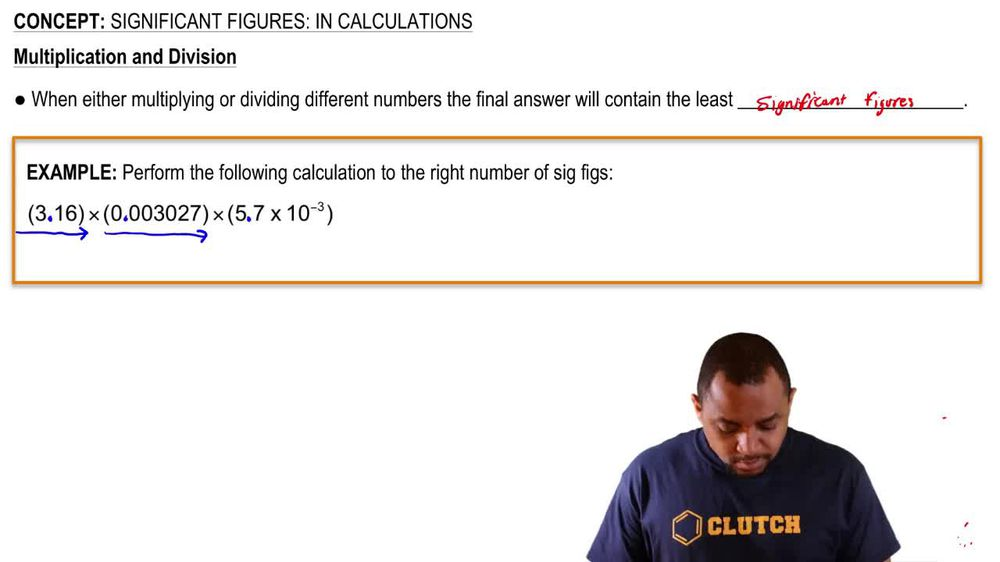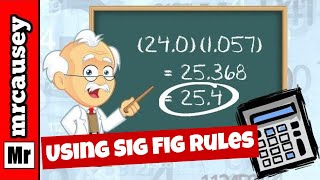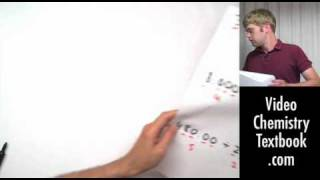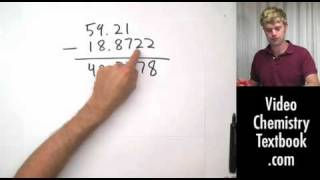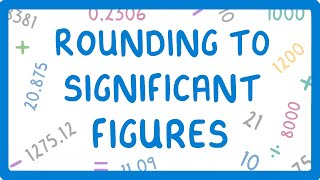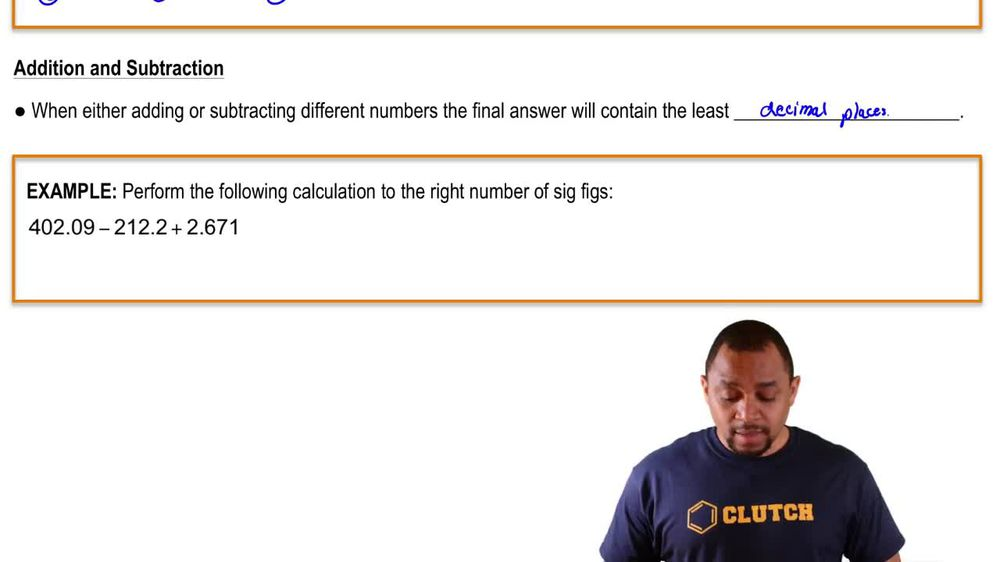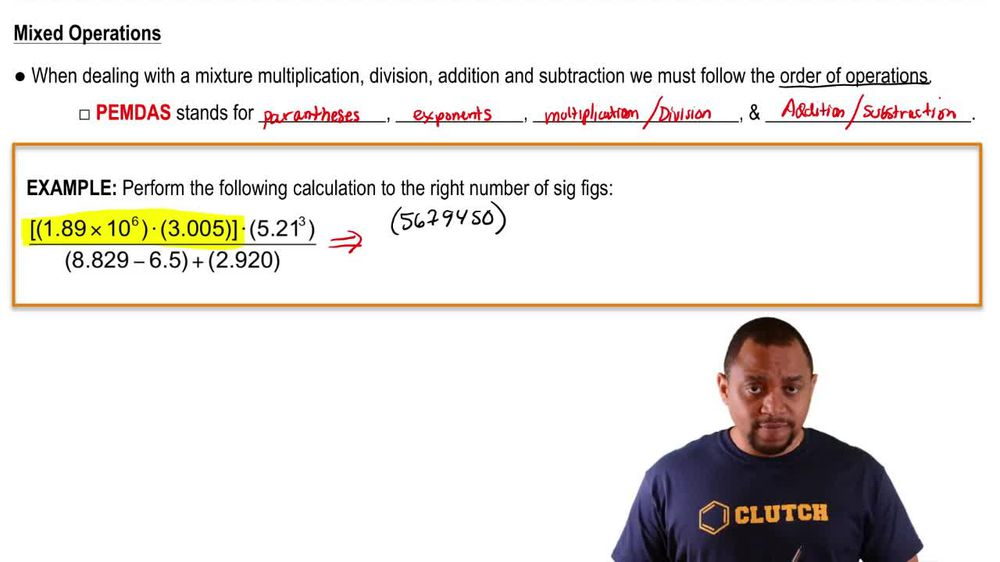Start typing, then use the up and down arrows to select an option from the list.
1. 1. Intro to General Chemistry2. Significant Figures: In Calculations
Problem

# Express the results of the following calculations with the correct number of significant figures. (c) 3.7 / 94.61

Relevant Solution1m
Play a video:
Hi everyone here. We have a question asking us to solve the problem using the correct number of significant figures In Parentheses. 43.1845, divided by 3.483. And out of parentheses divided by 0.249. So when we're doing multiplication and division, we want the least number of significant figures in this case. That is our 0.49 with three significant figures. Now let's rewrite our problem here. So we have 43 0. 45, divided by 3.483, divided by 0.249. And we're going to of course, solve what's in the parentheses first, which gives us 12 0.40, divided by 0.249. And that equals 49 0. 999. And we want three significant figures. So we're going to look at our seven here and we need to look at the right of it and nine is above five, So we will be rounding up. So our final answer will be 0.8. Thank you for watching. Bye.